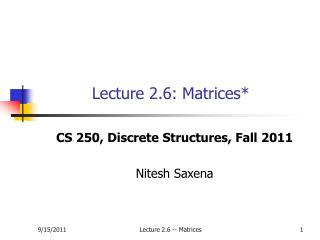DownloadDownload PresentationLecture 2.6: Matrices*

# Lecture 2.6: Matrices*

Download Presentation## Lecture 2.6: Matrices*

- - - - - - - - - - - - - - - - - - - - - - - - - - - E N D - - - - - - - - - - - - - - - - - - - - - - - - - - -
##### Presentation Transcript

1. Lecture 2.6: Matrices* CS 250, Discrete Structures, Fall 2011 Nitesh Saxena Lecture 2.6 -- Matrices

2. Course Admin • Mid-Term 1 on Thursday, Sep 22 • In-class (from 11am-12:15pm) • Will cover everything until the lecture on Sep 15 • No lecture on Sep 20 • As announced previously, I will be traveling to Beijing to attend and present a paper at the Ubicomp 2012 conference • This will not affect our overall topic coverage • This will also give you more time to prepare for the exam Lecture 2.6 -- Matrices

3. Course Admin • HW2 has been posted – due Sep 30 • Covers chapter 2 (lectures 2.*) • Start working on it, please. Will be helpful in preparation of the mid-term • HW1 grading delayed a bit • TA/grader was sick with chicken pox • Trying to finish as soon as possible • HW1 solution has been released Lecture 2.6 -- Matrices

4. Outline • Matrix • Types of Matrices • Matrix Operations Lecture 2.6 -- Matrices

5. Matrix – what it is? An array of numbers arranged in m horizontal rows and n vertical columns. We say that A is a matrix m x n. (Dimension of matrix) . A = {aij}, where i = 1, 2, …, m and j = 1, 2,…, n Lecture 2.6 -- Matrices

6. Examples • Grades obtained by a set of students in different courses can be represented a matrix • Average monthly temperature at a set of cities can be represented as a matrix • Facebook friend connections for a given set of users can be represented as a matrix • … Lecture 2.6 -- Matrices

7. Types of Matrices Square Matrix Number of rows = number of columns Which one(s)of the following is(are) squarematrix(ces)? Where is the main diagonal? Lecture 2.6 -- Matrices

8. Types of Matrices Diagonal Matrix “a square matrix in which entries outside the main diagonal area are all zero, the diagonal entries may or may not be zero” Lecture 2.6 -- Matrices

9. Equality of Matrices • Two matrices are said to be equal if the corresponding elements are equal. Matrix A = B iff aij = bij • Example: If A and B are equal matrices, find the values of a, b, x and y Lecture 2.6 -- Matrices

10. Equality of Matrices Find a, b, c, and d Find a, b, c, k, m, x, y, and z Equal Matrices - Work this out If 2. If Lecture 2.6 -- Matrices

11. Adding two Matrices Matrices Summation The sum of the matrices A and B is defined only when A and B have the same number of rows and the same number of columns (same dimension). C = A + B is defined as {aij + bij} Lecture 2.6 -- Matrices

12. Adding Two Matrices Matrices Summation – work this out a) Identify the pair of which matrices between which the summation process can be executed b) Compute C + G, A + D, E + H, A + F. Lecture 2.6 -- Matrices

13. Multiplying two Matrices • Steps before • Find out if it is possible to get the products? • Find out the result’s dimension • Arrange the numbers in an easy way to compute – avoid confusion Matrices Products Lecture 2.6 -- Matrices

14. Multiplying two Matrices Matrices Products – Possible outcomes Lecture 2.6 -- Matrices

15. Multiplying two Matrices Show that AB is NOT BA (this means that matrix multiplication is not commutative) Matrices Products – Work this out Let Lecture 2.6 -- Matrices

16. Matrix Transpose Transposition Matrix A matrix which is formed by turning all the rows of a given matrix into columns and vice-versa. The transpose of matrix A is written AT, and AT = {aji} Lecture 2.6 -- Matrices

17. Matric Transpose Compute (BA)T : Compute AT(D + F) Transposition Matrix – Work this out Lecture 2.6 -- Matrices

18. Symmetric Matrix Symmetrical Matrix A is said to be symmetric if all entries are symmetrical to its main diagonal. That is, if aij = aji Lecture 2.6 -- Matrices

19. Boolean Matrices Boolean Matrix and Its Operations • Boolean matrix is an m x n matrix where all of its entries are either 1 or 0 only. • There are three operations on Boolean: • Join by • Meet • Boolean Product Lecture 2.6 -- Matrices

20. Boolean Matrices Boolean Matrix and Its Operations – Join By Given A = [aij] and B = [bij] are Boolean matrices with the same dimension, join by A and B, written as A  B, will produce a matrix C = [cij], where cij = aij bij Lecture 2.6 -- Matrices

21. Boolean Matrices Boolean Matrix and Its Operations – Meet Meet for A and B, both with the same dimension, written as A  B, will produce matrix D = [dij] where dij = aij bij Lecture 2.6 -- Matrices

22. Boolean Matrices Boolean Matrix and Its Operations – Boolean Products If A = [aij] is an m x p Boolean matrix, and B = [bij] is a p x n Boolean matrix, we can get a Boolean product for A and B written as A ⊙ B, producing C, where: Lecture 2.6 -- Matrices

23. Boolean Matrices Lecture 2.6 -- Matrices

24. Boolean Matrices Work this out Lecture 2.6 -- Matrices

25. Today’s Reading • Rosen 2.6 Lecture 2.6 -- Matrices